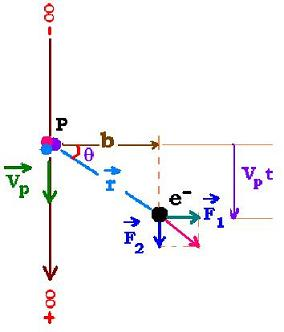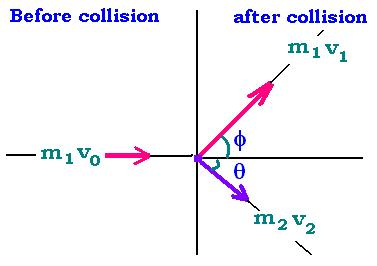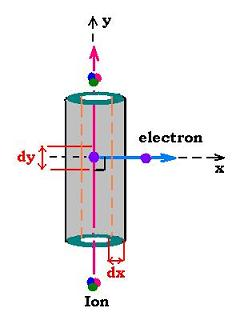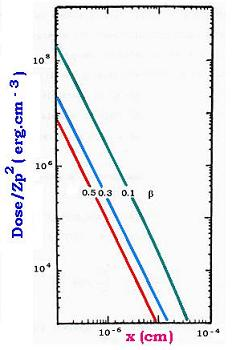Projectile's charge Z Projectile's E (MeV)

Contents

The nucleus

Statistical Mechanics

Related topics

### I. Classical Rutherford's differential cross section:Let's consider a collision between two particles Pp and Pc with charges Zp and Zc respectively. The related magnitude Coulomb's force is:
Fpc = (1/4πεo)ZpZc e2/r2 Where r is the distance between Pp and Pc at the time t. The perpendicular force to the Pp direction is the only important regarding the Coulomb's force. Fp becomes F = Fp cos θ = (1/4πεo)ZpZc e2 cos θ/r2.
The transferred moment to the particle Pc by Pp is: P = ∫Fdt.
r sin θ = Vp t → Vp dt = dr sinθ + b dθ.
Vp is the velocity of the Pp particle and b the impact parameter.
dr = b (sin θ/cos2 θ) dθ → Vp dt = (1 + tg2θ) dθ.
Then P = (1/4πεo)ZpZc e2 ∫ cos θ/r2 dt
= (1/4πεo)ZpZc e2 ∫ cos θ/r2 dt
= (1/4πεo)ZpZc e2 (1/Vpb) ∫ cos θ dθ [- π/2: +π/2].
P = ZpZc e2/2πεo Vpb    (1)

We consider the target particle Pc, that gains the transferred moment, is an electron of an atom of mass me. From the relationship (1), its energy becomes:
T = P2/2me = Zp2 e4/2me (2πεo Vpb)2    (2)
We solve the relationship (2) for b and derive over dT, we find the differential cross section dσ related to the collision :

dσ = 2πbdb = (2πZp2 eo4/mec2 β2) dT/T2    (3)

Where eo2 = e2/4πεo and β = Vp/C. c is the speed of light in the vacuum and e is the electron's charge = 1.6 10-19 Coulomb.
That is the differential cross section for that the projectile, with a charge Zp and velocity Vp, collides with an electron target of mass me and transferts to this electron a kinetic energy in the range T and T + dT.

### II: Binary Elastic collisionParticle 1: mass m1.
Particle 2: mass m2.

The conservation of energy and momentum before and after colision gives the following formulas:
m1v0 = m1v1 cosφ + m2v2 cosθ    (1)
m1v1 sinφ = m2v2 sinθ    (2)
m0v02 = m1 v12 + m2 v22    (3)

We square (1) and (2) and make the difference:
m12 v12 = m22 v22 sin2 θ + (m1v0 - m2v2 cos θ)2.
With the relationship (3), we find:
v2 = 2 ((m1/(m1 + m2)) v0 cosθ.

The kinetic energy tranferred to the second particle is: T = (1/2)m2v22; then:
We have:
T = 2 m12 m2 v02 cos2θ/(m1 + m2)2

cos2 θ = T/Tmax
Tmax = 2 m12 m2 v02/(m1 + m2)2

Tmax is the maximum energy that the particle 1 can transfert for the particle 2.

In the case of the particle 1 is more heavier than the second (m1 >> m2), we can write:
Tmax = 2 m2 v02.

Tmax (proton → electron) = 2 me Vp2

### III: The simplest approach: The Katz's model:In this model, an electron is ejected at low energy; that is cos &th�ta; is around zero degree.
If dn is the number of electrons ejected by the incident ion per unit length dy, and N is the number of electrons per unit volume, we have:
dn = N dσ = (C Zp22) dT/T2
C = 2πN eo4/me c2.
The distance-energy relationship:
We consider the following one:

x = k T(x)    (1)

From this relationship (1), we have xmax = kTmax and dT/T = k dx/x2

Where x is the diatance from the path of the incident ion, T(x) is the remaining enrgy of the electron at x, and k is a constant.
From the relationship (1), we get: dT/T2 = k dx/x2.

Only the electrons that have a kinetic energy T(x) or greater can arrive at x and traverse the layer dx, the number of those electrons is n(x) = ∫ dn(x) dx.
n(x) = (K Zp22) &int dT/T2.
= (C Zp22) [1/T(x) - 1/Tmax]
n(x) = k(C Zp22) [1/x - 1/xmax]    (2)

In the layer dx of volume dV = 2π x dx dy, where dy is the unit length of the ion's path, every electron deposits the enrgy dT = dx/k; forthermore n(x) electrons crossing the layer dx will deposit in the volume dV the energy n(x) dT = n(x) dx/k.
The dose defined as the enery deposoited by volume unit is then written as:
D(x,βCp) = (n(x) dx/k)/dV = (n(x) dx/k)/(2π x dx dy). From the relationship (2), we can write:
= D(x,βCp) = k(C Zp22) [1/x - 1/xmax] dx/k /(2π x dx dy)
= (C Zp22) [1/x - 1/xmax] /(2π x dy)
Generally, xmax is often greater than x, we have then, per unit length of the incomming ion:

D(x,&beta,Zp) = [C Zp2/2π β2] 1/x2    (3)For 100 MeV protons in water, we have:
N = 3.35 x 10-23
C = 8.50 (e-) eV/µm
Energy of proton Ep = (1/2) MpVp2
= (1/2) Mpc2 β2 = (1/2) 938.272 β2 MeV = 469.136 β2 MeV
Ep = 469.136 β2 MeV
With Ep = 100 MeV → β = 0.462
D(x,100 meV) = [8.50 eV/µm /2π β2] 1/x2 = 63.50 1/x2 keV/cm3

D(x,100 MeV) = 63.50/x2 keV.cm- 3

In erg unit, we have:
D(x,100 meV) = 1.018 10 - 7/ x2 erg . cm-3Web ScientificSentence